# 5.3 The Range of a Sinusoidal Function

Handout: FOM 12 5.3 Determine the Range

The range of the graphisThe two tranformations we can make to thevalues are to

• multiply (or divide)

In general, a sinusoidal graph has equation. It is only the valuesandthat alter the range of the graph.

# Multiply

To draw the graph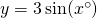we consider particular points (easy points), and multiply thecoordinate by 3 as follows: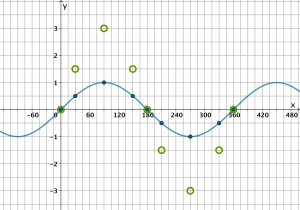Track each point in turn. For example, on the blue line we have the pointtherefore we plot the new point. Thecoordinate (that is,) is multiplied by.

Next we draw a line through our new points: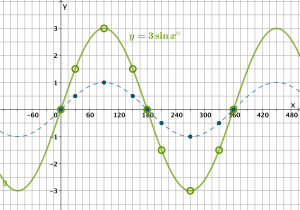We see that the range of the green curve is.

The amplitude of this curve is.

The sinusoidal axis is not changed, it is still.

To draw the graphagain we consider particular points and add 2 to thevalue as follows:As before, track each point in turn. For example, the point on the blue curve atwill become the point.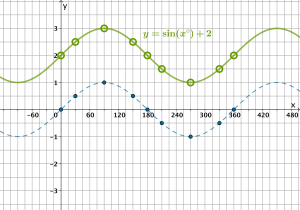We see that the range of the green curve is.

The sinusoidal axis is the horizontal line.

The amplitude of the curve is not changed, it is still 1.

# Try each transformation here:

To do both operations, we should multiply first then add. However, in practice it is easier to draw a new sinusoidal axis, and plot the correct amplitude from there.

For example, transform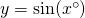to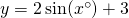.

First, lets draw a new sinusoidal axis atNow let’s find the multiples of 180 on the lineto plot our new ‘zeros’: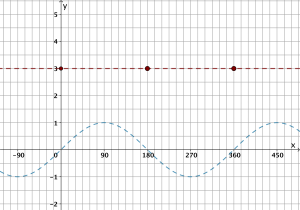Now let’s track the multiples of 90, and plot our new max and min but remembering that the amplitude ofis 3, so we plot 3 above and below the sinusoidal axis: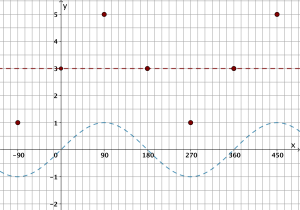Finally, we can draw our curve and erase the sinusoidal axis:The range of our new graph is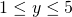, which we can see is the same as.

In general, we can say that the range of the sinusoidal functionis(whenis positive, otherwise the inequality is reversed).

Try both transformations together here:

Practice: Determine the range

CA1 Test out: Determine the range accuracy quiz

Practice: Match the graph

CA2 Test out: Match the graph